Vectors - Motion and Forces in Two Dimensions - Lesson 3 - Forces in Two Dimensions

# Net Force Problems Revisited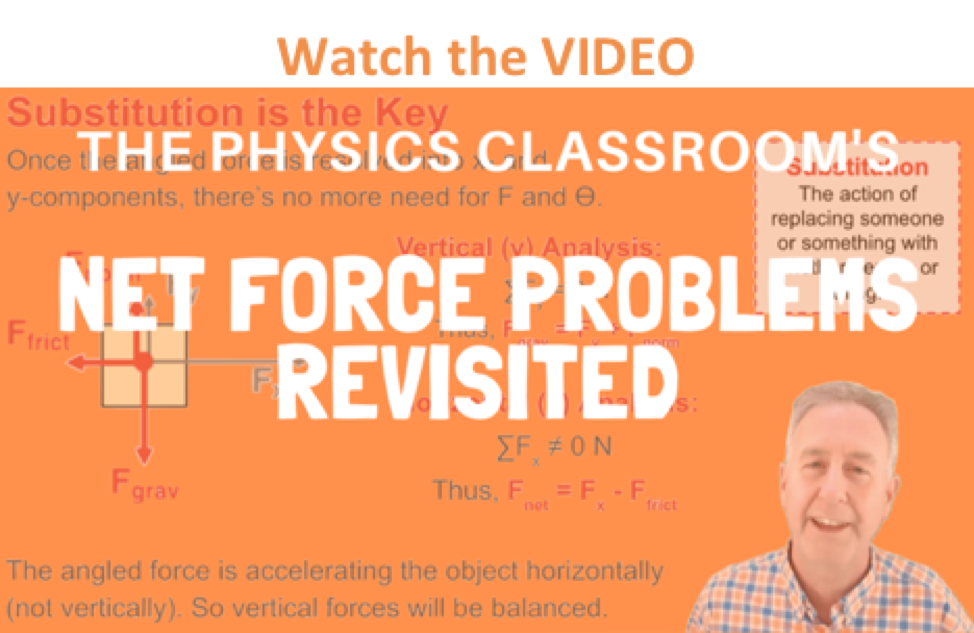This part of Lesson 3 focuses on net force-acceleration problems in which an applied force is directed at an angle to the horizontal. We have already discussed earlier in Lesson 3 how a force directed an angle can be resolved into two components - a horizontal and a vertical component. We have also discussed in an earlier unit that the acceleration of an object is related to the net force acting upon the object and the mass of the object (Newton's second law). We had used this principle to solve net force-acceleration problems in an earlier unit. Therefore, it is a natural extension of this unit to combine our understanding of Newton's second law with our understanding of force vectors directed at angles.

### Simplifying a Difficult Problem

Consider the situation below in which a force is directed at an angle to the horizontal. In such a situation, the applied force could be resolved into two components. These two components can be considered to replace the applied force at an angle. By doing so, the situation simplifies into a familiar situation in which all the forces are directed horizontally and vertically.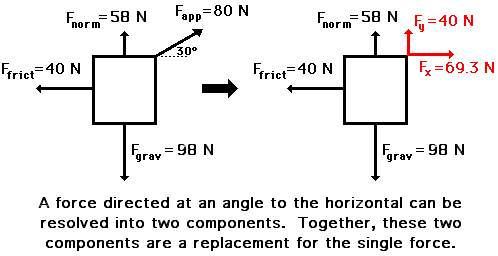Once the situation has been simplified, the problem can be solved like any other problem. The task of determining the acceleration involves first determining the net force by adding up all the forces as vectors and then dividing the net force by the mass to determine the acceleration. In the above situation, the vertical forces are balanced (i.e., Fgrav, Fy, and Fnorm add up to 0 N), and the horizontal forces add up to 29.3 N, right (i.e., 69.3 N, right + 40 N, left = 29.3 N, right). The net force is 29.3 N, right and the mass is 10 kg (m = Fgrav/g); therefore, the acceleration is 2.93 m/s/s, right.

To test your understanding, analyze the two situations below to determine the net force and the acceleration. When finished, click the button to view the answers.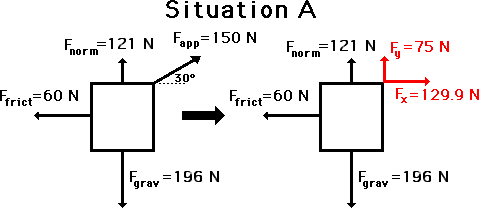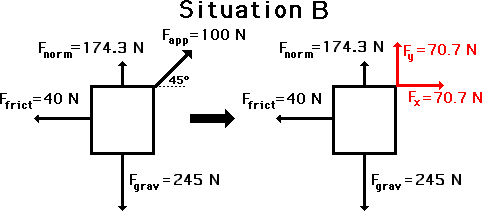### What's Up with the Normal Force?

There is one peculiarity about these types of problems that you need to be aware of. The normal force (Fnorm) is not necessarily equal to the gravitational force (Fgrav) as it has been in problems that we have previously seen. The principle is that the vertical forces must balance if there is no vertical acceleration. If an object is being dragged across a horizontal surface, then there is no vertical acceleration. For this reason, the normal force (Fnorm) plus the vertical component (Fy) of the applied force must balance the gravitational force (Fgrav). A quick review of these problems shows that this is the case. If there is an acceleration for an object being pulled across a floor, then it is a horizontal acceleration; and thus the only imbalance of force would be in the horizontal direction.

Now consider the following situation in which a force analysis must be conducted to fill in all the blanks and to determine the net force and acceleration. In a case such as this, a thorough understanding of the relationships between the various quantities must be fully understood. Make an effort to solve this problem. When finished, click the button to view the answers. (When you run into difficulties, consult the help from a previous unit.)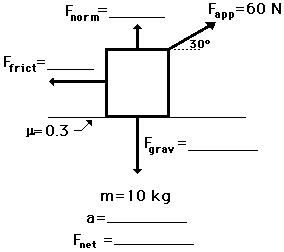In conclusion, a situation involving a force at an angle can be simplified by using trigonometric relations to resolve that force into two components. Such a situation can be analyzed like any other situation involving individual forces. The net force can be determined by adding all the forces as vectors and the acceleration can be determined as the ratio of Fnet/mass.

## freestar.config.enabled_slots.push({ placementName: "physicsclassroom_incontent_3", slotId: "physicsclassroom_incontent_3" });

The following problems provide plenty of practice with Fnet= m • a problems involving forces at angles. Try each problem and then click the button to view the answers.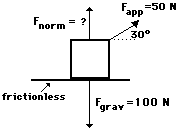1. A 50-N applied force (30 degrees to the horizontal) accelerates a box across a horizontal sheet of ice (see diagram). Glen Brook, Olive N. Glenveau, and Warren Peace are discussing the problem. Glen suggests that the normal force is 50 N; Olive suggests that the normal force in the diagram is 75 N; and Warren suggests that the normal force is 100 N. While all three answers may seem reasonable, only one is correct. Indicate which two answers are wrong and explain why they are wrong.

2. A box is pulled at a constant speed of 0.40 m/s across a frictional surface. Perform an extensive analysis of the diagram below to determine the values for the blanks.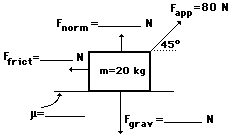3. Use your understanding of force relationships and vector components to fill in the blanks in the following diagram and to determine the net force and acceleration of the object. (Fnet = m•a; Ffrict = μ•Fnorm; Fgrav = m•g)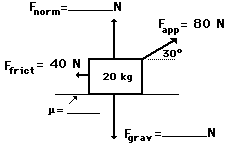4. The 5-kg mass below is moving with a constant speed of 4 m/s to the right. Use your understanding of force relationships and vector components to fill in the blanks in the following diagram and to determine the net force and acceleration of the object. (Fnet = m•a; Ffrict = μ•Fnorm; Fgrav = m•g)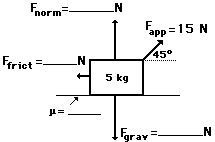5. The following object is being pulled at a constant speed of 2.5 m/s. Use your understanding of force relationships and vector components to fill in the blanks in the following diagram and to determine the net force and acceleration of the object. (Fnet = m•a; Ffrict = μ•Fnorm; Fgrav = m•g)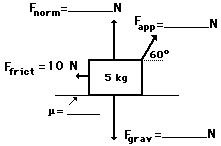6. Use your understanding of force relationships and vector components to fill in the blanks in the following diagram and to determine the net force and acceleration of the object. (Fnet = m•a; Ffrict = μ•Fnorm; Fgrav = m•g)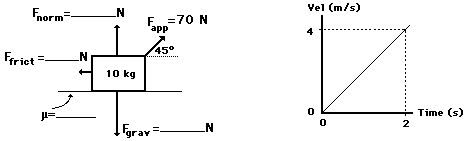7. Study the diagram below and determine the acceleration of the box and its velocity after being pulled by the applied force for 2.0 seconds.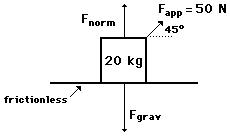8. A student pulls a 2-kg backpack across the ice (assume friction-free) by pulling at a 30-degree angle to the horizontal. The velocity-time graph for the motion is shown. Perform a careful analysis of the situation and determine the applied force.9. The following object is moving to the right and encountering the following forces. Use your understanding of force relationships and vector components to fill in the blanks in the following diagram and to determine the net force and acceleration of the object. (Fnet = m•a; Ffrict = μ•Fnorm; Fgrav = m•g)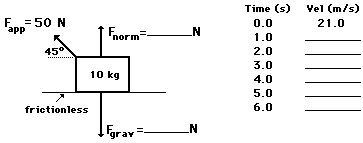10. The 10-kg object is being pulled to the left at a constant speed of 2.5 m/s. Use your understanding of force relationships and vector components to fill in the blanks in the following diagram. (Fnet = m•a; Ffrict = μ•Fnorm; Fgrav = m•g)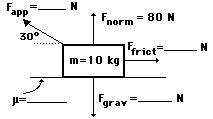11. Use your understanding of force relationships and vector components to fill in the blanks in the following diagram and to determine the net force and acceleration of the object. (Fnet = m•a; Ffrict = μ•Fnorm; Fgrav = m•g)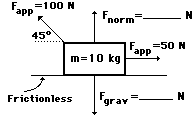Next Section: